﻿ Python: Circular shift number - w3resource# Python: Circular shift number

## Python Programming Puzzles: Exercise-65 with Solution

Write a Python program to shift the decimal digits n places to the left, wrapping the extra digits around. If shift > the number of digits of n, reverse the string.

```Input:
n = 12345  and shift = 1
Output:
Result =  23451
Input:
n = 12345  and shift = 2
Output:
Result =  34512
Input:
n = 12345  and shift = 3
Output:
Result =  45123
Input:
n = 12345  and shift = 5
Output:
Result =  12345
Input:
n = 12345  and shift = 6
Output:
Result =  54321
```

Pictorial Presentation: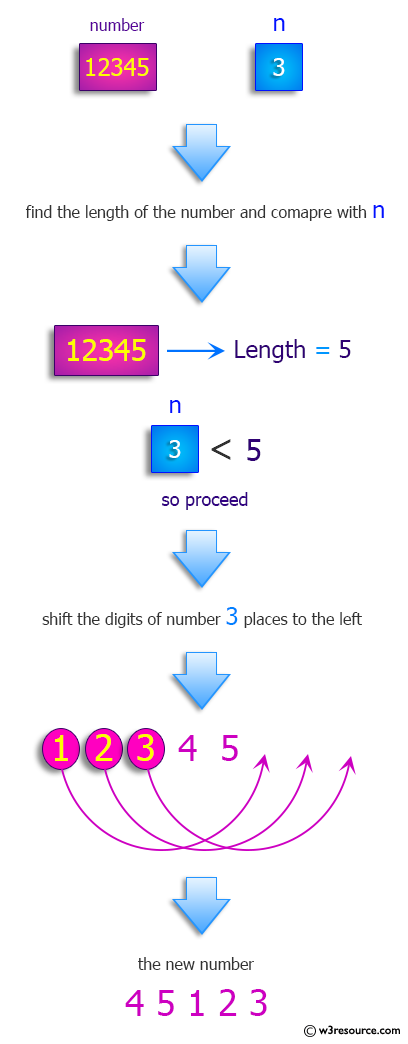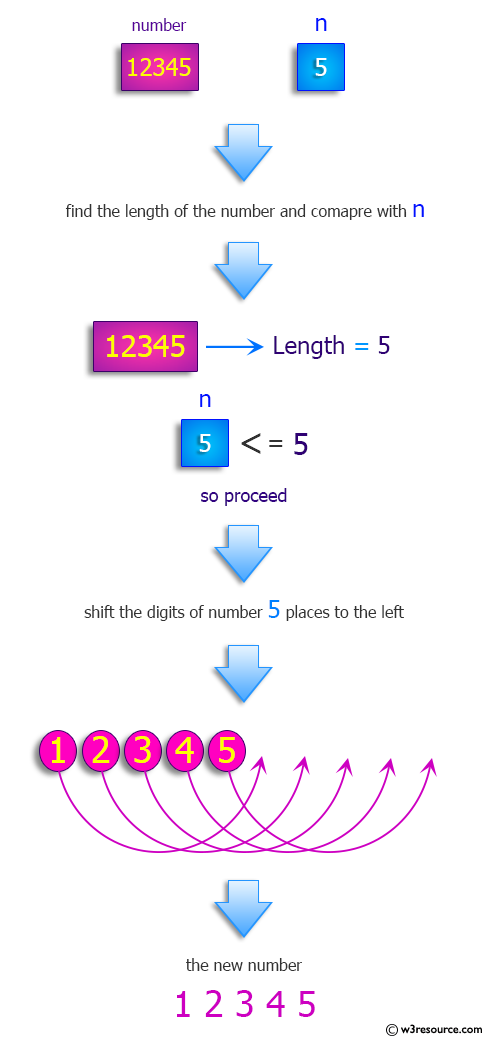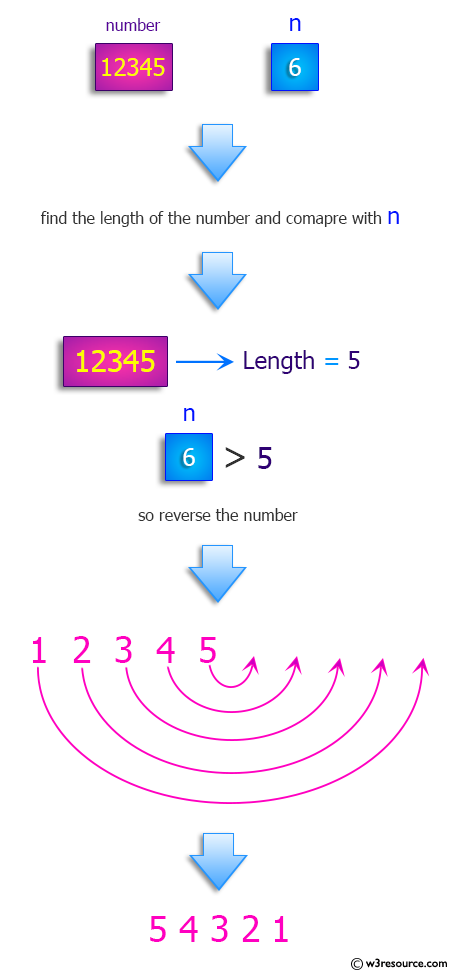Sample Solution-1:

Python Code:

``````#License: https://bit.ly/3oLErEI

def test(n, shift):
s = str(n)
if shift > len(s):
return s[::-1]
return s[shift:] + s[:shift]

print("Shift the decimal digits n places to the left. If shift > the number of digits of n, reverse the string.:")

n = 12345
shift = 1
print("\nn =",n," and shift =",shift)
print("Result = ",test(n, shift))
n = 12345
shift = 2
print("\nn =",n," and shift =",shift)
print("Result = ",test(n, shift))
n = 12345
shift = 3
print("\nn =",n," and shift =",shift)
print("Result = ",test(n, shift))
n = 12345
shift = 5
print("\nn =",n," and shift =",shift)
print("Result = ",test(n, shift))
n = 12345
shift = 6
print("\nn =",n," and shift =",shift)
print("Result = ",test(n, shift))
``````

Sample Output:

```Shift the decimal digits n places to the left. If shift > the number of digits of n, reverse the string.:

n = 12345  and shift = 1
Result =  23451

n = 12345  and shift = 2
Result =  34512

n = 12345  and shift = 3
Result =  45123

n = 12345  and shift = 5
Result =  12345

n = 12345  and shift = 6
Result =  54321
```

Flowchart: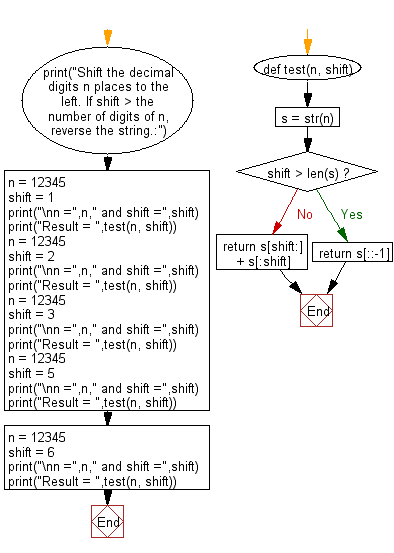## Visualize Python code execution:

The following tool visualize what the computer is doing step-by-step as it executes the said program:

Sample Solution-2:

Python Code:

``````#License: https://bit.ly/3oLErEI

def test(n, shift):
shifted_digits = [int(x) for x in str(n)]
for i in range(shift):
shifted_digits.append(shifted_digits.pop(0))
if shift > len(shifted_digits):
return str(n)[::-1]
else:
return ''.join(str(x) for x in shifted_digits)

print("Shift the decimal digits n places to the left. If shift > the number of digits of n, reverse the string.:")

n = 12345
shift = 1
print("\nn =",n," and shift =",shift)
print("Result = ",test(n, shift))
n = 12345
shift = 2
print("\nn =",n," and shift =",shift)
print("Result = ",test(n, shift))
n = 12345
shift = 3
print("\nn =",n," and shift =",shift)
print("Result = ",test(n, shift))
n = 12345
shift = 5
print("\nn =",n," and shift =",shift)
print("Result = ",test(n, shift))
n = 12345
shift = 6
print("\nn =",n," and shift =",shift)
print("Result = ",test(n, shift))
``````

Sample Output:

```Shift the decimal digits n places to the left. If shift > the number of digits of n, reverse the string.:

n = 12345  and shift = 1
Result =  23451

n = 12345  and shift = 2
Result =  34512

n = 12345  and shift = 3
Result =  45123

n = 12345  and shift = 5
Result =  12345

n = 12345  and shift = 6
Result =  54321
```

Flowchart: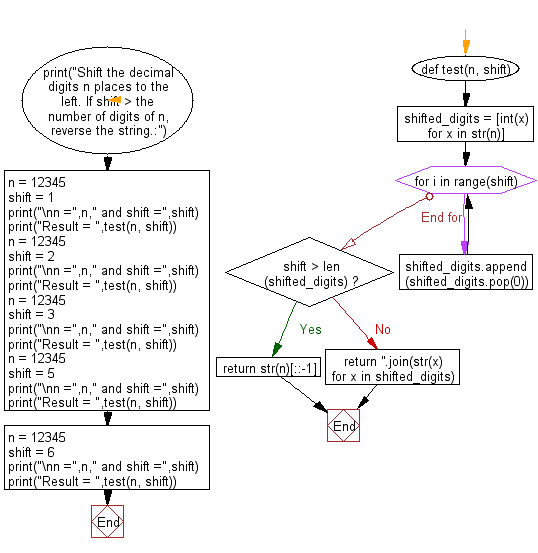## Visualize Python code execution:

The following tool visualize what the computer is doing step-by-step as it executes the said program:

Python Code Editor :

Have another way to solve this solution? Contribute your code (and comments) through Disqus.

What is the difficulty level of this exercise?

Test your Programming skills with w3resource's quiz.

﻿

## Python: Tips of the Day

Clamps num within the inclusive range specified by the boundary values x and y:

Example:

```def tips_clamp_num(num,x,y):
return max(min(num, max(x, y)), min(x, y))
print(tips_clamp_num(2, 4, 6))
print(tips_clamp_num(1, -1, -6))
```

Output:

```4
-1
```